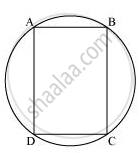# Prove that a Cyclic Parallelogram is a Rectangle. - Mathematics

Prove that a cyclic parallelogram is a rectangle.

#### SolutionLet ABCD be a cyclic parallelogram.

∠A + ∠C = 180° (Opposite angles of a cyclic quadrilateral) ... (1)

We know that opposite angles of a parallelogram are equal.

∴ ∠A = ∠C and ∠B = ∠D

From equation (1),

∠A + ∠C = 180°

⇒ ∠A + ∠A = 180°

⇒ 2 ∠A = 180°

⇒ ∠A = 90°

Parallelogram ABCD has one of its interior angles as 90°. Therefore, it is a rectangle.

Is there an error in this question or solution?

#### APPEARS IN

NCERT Class 9 Maths
Chapter 10 Circles
Exercise 10.5 | Q 12 | Page 186